# Minimal set

(diff) ← Older revision | Latest revision (diff) | Newer revision → (diff)
A minimal set in a Riemannian space is a generalization of a minimal surface. A minimal set is a-dimensional closed subsetin a Riemannian space,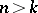, such that for some subset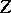of-dimensional Hausdorff measure zero the set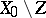is a differentiable-dimensional minimal surface (that is, is an extremum of the-dimensional volume functional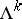, defined on-dimensional surfaces imbedded in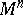). The notion of a "minimal set" amalgamates several mathematical ideas called upon to serve in the so-called multi-dimensional Plateau problem (cf. also Plateau problem, multi-dimensional).
A minimal set in a topological dynamical system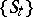is a non-empty closed invariant (that is, consisting wholly of trajectories) subsetof the phase spaceof the system which does not have proper closed invariant subsets. The latter is equivalent to saying that each trajectory inis everywhere dense in. The notion of a minimal set was introduced by G.D. Birkhoff (see ) for the case of a flow (the "time"running through the real numbers). He proved (see , ) that ifis a compact minimal set and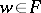, then for any neighbourhoodof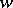the set of thosefor which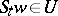is relatively dense in(that is, there is ansuch that in each "time interval"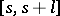of lengththere is at least onewith); conversely, ifis a complete metric space and a pointhas the above property, then the closure of its trajectoryis a compact minimal set (the same is true for a cascade; regarding more general groups of transformations see, for example,  and ). Birkhoff called this property of(and its trajectory) recurrence; another terminology, suggested by W.H. Gottschalk and G.A. Hedlund , is also used, in which this property is called almost-periodicity of the point. If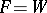, then the dynamical system itself is called minimal.
If a trajectory has compact closure, then it contains a minimal set(for semi-groups of continuous transformations, with non-negative real or integer, an analogue of this result holds, where inthe transformations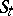are even invertible ). However, research into the limit behaviour of the trajectories of a dynamical system does not reduce to the study of only the minimal sets of the latter. A minimal set of a smooth flow of class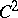on a two-dimensional closed surfacehas a very simple structure: it is either a point, a closed trajectory or the whole surface, which is then a torus (Schwarz's theorem, ). In the general case the structure of a minimal set can be very complicated (in this connection, in addition to what is said in  it must be said that minimality of a dynamical system places no restrictions on its ergodic properties with respect to any of its invariant measures, ). Minimal sets are the fundamental objects of study in topological dynamics.